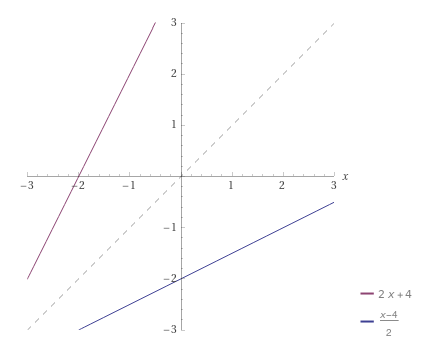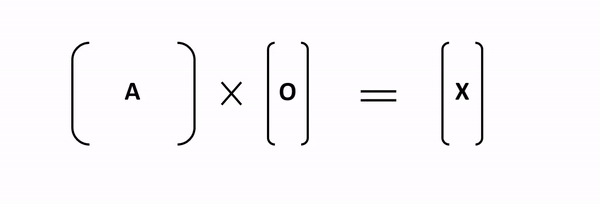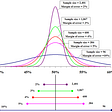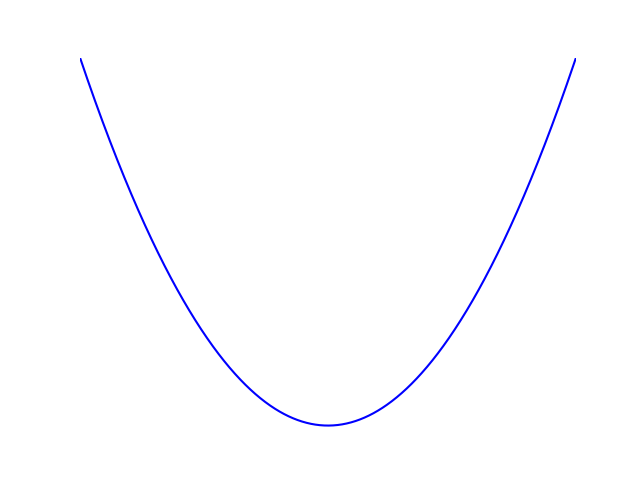# How to Find the Inverse Function

To find the inverse function, follow these four steps:

1. Write the equation as a linear equation (swap `f(x)` with `y`).
2. Swap variables `x` and `y`.
3. Solve for `y`.
4. Apply the inverse notation (replace `y` with `f^(-1)(x)`).

# Example

Find the inverse function of

Solution. Let’s apply the above steps.

1. Write the equation as a linear equation.

2. Swap variables `x` and `y`.

3. Solve for `y`.

Subtract `4` from both sides:

Divide both sides by `2`:

You can simplify the left-hand side by separately dividing both values of the numerator:

4. Apply the inverse notation:

If you want, you can use a tool like WolframAlpha to plot your functions:The function and the inverse are each other’s mirror images along the x = y line.

--

--

--

## More from Artturi Jalli

I’m a tech expert, entrepreneur, and software engineer from Finland. I write about programming, tech, and science. Visit my blog at www.codingem.com.

Love podcasts or audiobooks? Learn on the go with our new app.

## A Simple Mathematical problem that is unsolved## Maths Hack: Easily Compute the Power of a Matrix using Diagonalization## American mathematics contest 8## Confidence Interval and Sample Size## The Fano Plane — Hloni Introduction## A Practical Math Problem## Artturi Jalli

I’m a tech expert, entrepreneur, and software engineer from Finland. I write about programming, tech, and science. Visit my blog at www.codingem.com.

## How Far Is the Horizon## Rotating a graph around the y-axis - How would we see it on an x-y plane?## A Rebel or Forced to Rebel?